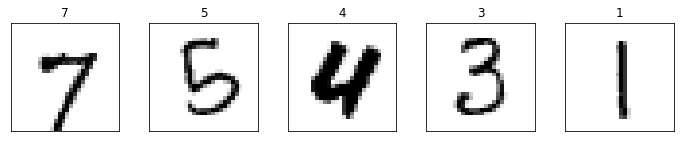# MNIST Using Keras

In this notebook, we will build a simple two-layer feed-forward neural network model using Keras, running on top of TensorFlow. We then train the sequential model using 60,000 MNIST digits and evaluate it on 10,000 MNIST digits.

I put this notebook together to briefly comment the code from chapter 2 of François Chollet’s excellent book, Deep Learning with Python.

import matplotlib.pyplot as plt
import numpy as np
from keras.datasets import mnist
from keras import models, layers
from keras.utils import to_categorical

np.random.seed(22)

Using TensorFlow backend.


Each digit is a monochrome 28 by 28 pixels image. The training set consists of 60,000 images and the testing set of 10,000 images. Each image in the training and testing set has a corresponding label provided, indicating the true value of the digit in the image.

(train_images, train_labels), (test_images, test_labels) = mnist.load_data()


## Training and Testing Data Shape and Type

print(train_images.shape)
print(len(train_labels))
print("First 10 labels: {0} -> {1}".format(train_labels[:10], type(train_labels)))

(60000, 28, 28)
60000
First 10 labels: [5 0 4 1 9 2 1 3 1 4] -> <class 'numpy.uint8'>

print(test_images.shape)
print(len(test_labels))
print("First 10 labels: {0} -> {1}".format(test_labels[:10], type(test_labels)))

(10000, 28, 28)
10000
First 10 labels: [7 2 1 0 4 1 4 9 5 9] -> <class 'numpy.uint8'>


## Displaying Random Samples from Training Digits

num_plot_digits = 5
digits_to_plot = np.random.randint(0, 60000, num_plot_digits)
fig, axes = plt.subplots(1, 5, figsize=(12,2))

for i in range(num_plot_digits):
axes[i].imshow(train_images[digits_to_plot[i]], cmap=plt.cm.binary)
axes[i].set_title(train_labels[digits_to_plot[i]])
axes[i].set_xticks([])
axes[i].set_yticks([])## Network Architecture

Two fully connected (dense) layers, with the first layer using ReLU for activation and the second (last/output) layer using softmax.

network = models.Sequential()
network.add(layers.Dense(512, activation='relu', input_shape=(28 * 28, )))
network.compile(optimizer='rmsprop',
loss='categorical_crossentropy',
metrics=['accuracy'])


## Preprocessing Data

The data will be reshaped so that each sample image is a row 784 columns long (28 * 28), as expected by the network. Furthermore, the data will be normalized so all values are in the [0,1] interval and their type changed to float32 from uint8.

The labels will in turn be converted to a categorical type, i.e. one-hot encoded.

train_images = train_images.reshape((60000, 28 * 28))
train_images = train_images.astype('float32') / 255

test_images = test_images.reshape((10000, 28 * 28))
test_images = test_images.astype('float32') / 255

train_labels = to_categorical(train_labels)
test_labels = to_categorical(test_labels)


## Training the Network

Fitting the model to the training data using 5 epochs and a batch size of 128.

network.fit(train_images, train_labels, epochs=5, batch_size=128)

Epoch 1/5
60000/60000 [==============================] - 15s 254us/step - loss: 0.2599 - acc: 0.9238
Epoch 2/5
60000/60000 [==============================] - 15s 257us/step - loss: 0.1045 - acc: 0.9691
Epoch 3/5
60000/60000 [==============================] - 15s 258us/step - loss: 0.0695 - acc: 0.9790
Epoch 4/5
60000/60000 [==============================] - 16s 263us/step - loss: 0.0501 - acc: 0.9847
Epoch 5/5
60000/60000 [==============================] - 16s 264us/step - loss: 0.0379 - acc: 0.9886

<keras.callbacks.History at 0x7fe2d3a750b8>


## Testing the Network

Testing the accuracy of the fitted model on the testing data set.

test_loss, test_acc = network.evaluate(test_images, test_labels)
print('loss: {0:.4f} - acc: {1:.4f}'.format(test_loss, test_acc))

10000/10000 [==============================] - 2s 192us/step
loss: 0.0651 - acc: 0.9806


## Conclusion

This simple two-layer dense sequential network manages an accuracy of 98.86% on the training data set and 98.06% on the testing data set. Much better results can be achieved, well above 99% accuracy, using various ways. For instance, convolutional neural networks. Refer to Yann LeCun’s MNIST page for details of other approaches and the test error rate achieved.

Tags:

Categories:

Updated: